## Introduction

This document shows the basics of applying our Bayesian model-based clustering/classification with joint batch correction in `R`. It shows how to generate some toy data, apply the model, assess convergence and process outputs.

## Data generation

We simulate some data using the `generateBatchData` function.

``````library(ggplot2)
library(batchmix)

# Data dimensions
N <- 600
P <- 4
K <- 5
B <- 7

# Generating model parameters
mean_dist <- 2.25
batch_dist <- 0.3
group_means <- seq(1, K) * mean_dist
batch_shift <- rnorm(B, mean = batch_dist, sd = batch_dist)
std_dev <- rep(2, K)
batch_var <- rep(1.2, B)
group_weights <- rep(1 / K, K)
batch_weights <- rep(1 / B, B)
dfs <- c(4, 7, 15, 60, 120)

my_data <- generateBatchDataMVT(
N,
P,
group_means,
std_dev,
batch_shift,
batch_var,
group_weights,
batch_weights,
dfs
)
``````

This gives us a named list with two related datasets, the `observed_data` which includes batch effects and the `corrected_data` which is batch-free. It also includes `group_IDs`, a vector indicating class membership for each item, `batch_IDs`, which indicates batch of origin for each item, and `fixed`, which indicates which labels are observed and fixed in the model. We pull these out of the names list in the format that the modelling functions desire them.

``````X <- my_data\$observed_data

true_labels <- my_data\$group_IDs
fixed <- my_data\$fixed
batch_vec <- my_data\$batch_IDs

alpha <- 1
initial_labels <- generateInitialLabels(alpha, K, fixed, true_labels)
``````

## Modelling

Given some data, we are interested in modelling it. We assume here that the set of observed labels includes at least one example of each class in the data.

``````# Sampling parameters
R <- 1000
thin <- 50
n_chains <- 4

# Density choice
type <- "MVT"

# MCMC samples and BIC vector
mcmc_output <- runMCMCChains(
X,
n_chains,
R,
thin,
batch_vec,
type,
initial_labels = initial_labels,
fixed = fixed
)
``````

We want to assess two things. First, how frequently the proposed parameters in the Metropolis-Hastings step are accepted:

``````plotAcceptanceRates(mcmc_output)
``````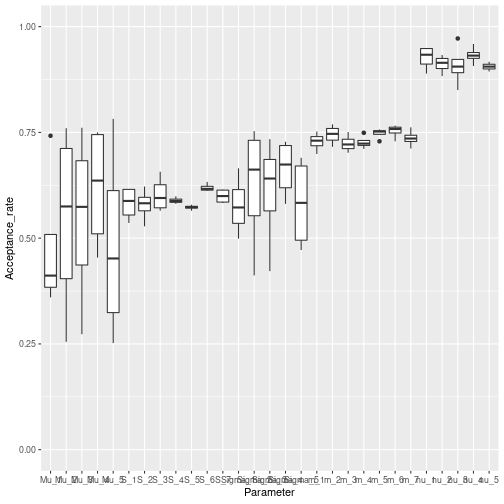Secondly, we want to asses how well our chains have converged. To do this we plot the `complete_likelihood` of each chain. This is the quantity most relevant to a clustering/classification, being dependent on the labels. The `observed_likelihood` is independent of labels and more relevant for density estimation.

``````plotLikelihoods(mcmc_output)
``````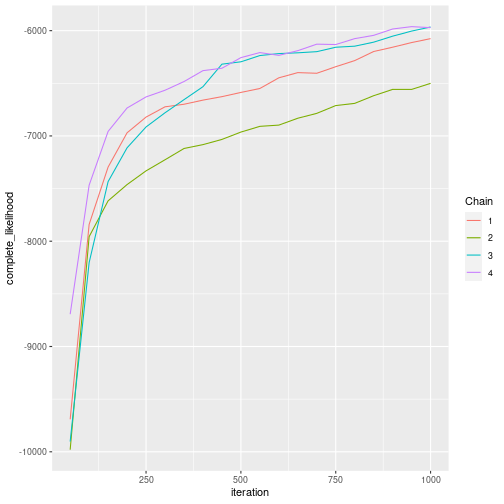We see that our chains disagree. We have to run them for more iterations. We use the `continueChains` function for this.

``````R_new <- 9000

# Given an initial value for the parameters
new_output <- continueChains(
mcmc_output,
X,
fixed,
batch_vec,
R_new,
keep_old_samples = TRUE
)
``````

To see if the chains better agree we re-plot the likelihood.

``````plotLikelihoods(new_output)
``````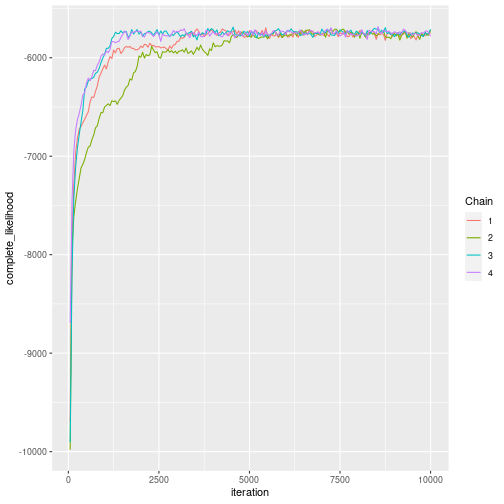We also re-check the acceptance rates.

``````plotAcceptanceRates(new_output)
``````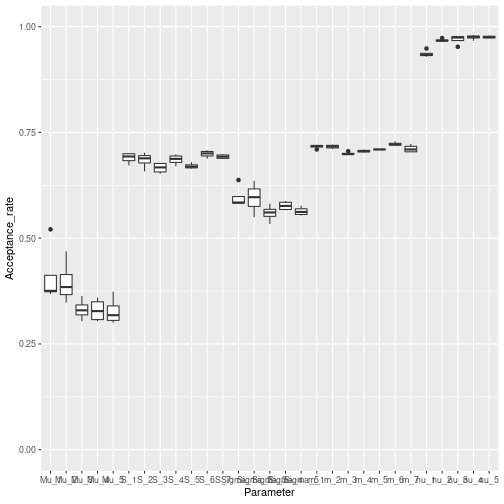This looks like several of the chains agree by the 5,000th iteration.

## Process chains

We process the chains, acquiring point estimates of different quantities.

``````# Burn in
burn <- 5000

# Process the MCMC samples
processed_samples <- processMCMCChains(new_output, burn)
``````

## Visualisation

For multidimensional data we use a PCA plot.

``````chain_used <- processed_samples[]

pc <- prcomp(X, scale = T)
pc_batch_corrected <- prcomp(chain_used\$inferred_dataset)

plot_df <- data.frame(
PC1 = pc\$x[, 1],
PC2 = pc\$x[, 2],
PC1_bf = pc_batch_corrected\$x[, 1],
PC2_bf = pc_batch_corrected\$x[, 2],
pred_labels = factor(chain_used\$pred),
true_labels = factor(true_labels),
prob = chain_used\$prob,
batch = factor(batch_vec)
)

plot_df |>
ggplot(aes(
x = PC1,
y = PC2,
colour = true_labels,
alpha = prob
)) +
geom_point()
``````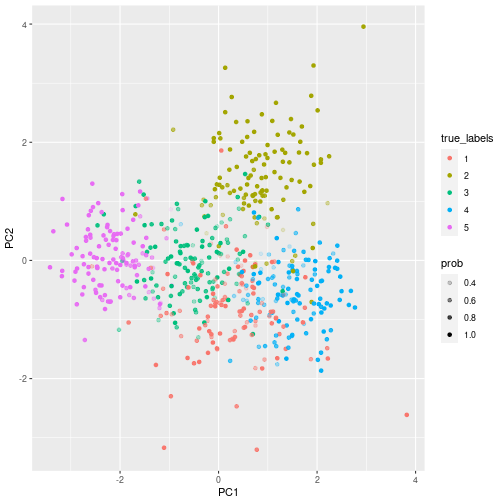``````plot_df |>
ggplot(aes(
x = PC1_bf,
y = PC2_bf,
colour = pred_labels,
alpha = prob
)) +
geom_point()
``````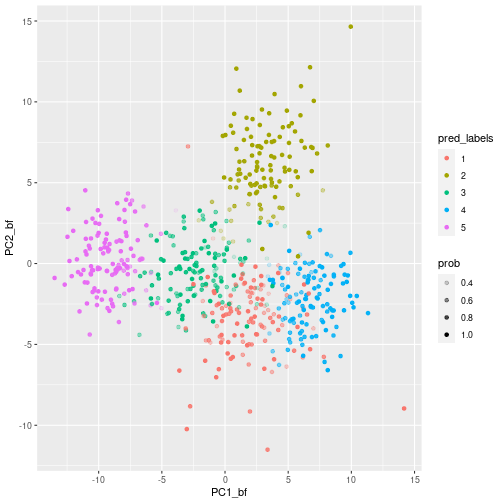``````test_inds <- which(fixed == 0)

sum(true_labels[test_inds] == chain_used\$pred[test_inds])/length(test_inds)
``````
``````##  0.7957447
``````